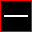# Grey Olltwit's Maths Worksheet - Subtraction

2.2.0.94

Grey Olltwit's Maths Worksheet - Subtration is a simple application which can help to develop your kids' math skills. Choose from 3 levels of subtraction sums from single digits to three figure problems. Each sum is randomly produced one at a time on a blackboard style interface.

Commercial \$19.95
Date:
Category rank:
1 527
All software rank:
62 895

## More about Grey Olltwit's Maths Worksheet - Subtraction

The commercial software of Grey Olltwit Educational Software comes with the \$19.95 price tag. Grey Olltwit's Maths Worksheet - Subtraction belongs to the Education category, Science. Subtract.exe is the most popular setup file of this program. The antivirus check of the download link proved it to be clean.Download
Grey Olltwit's Maths Worksheet - Subtraction 2.2.0.94

## Comments on Grey Olltwit's Maths Worksheet - Subtraction

Thank you for rating the program!

### Useful software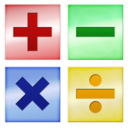Microsoft Math Worksheet Generator
Microsoft Math Worksheet Generator creates multiple math practice problems.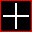Grey Olltwit's Maths Worksheet - Addition
It is a simple application which can help to develop your kids' math skills.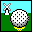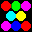Grey Olltwit's Marble Buster
This is a breakout type game where coloured marbles descend towards you.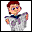Grey Olltwit's Social Stories
This program contains a number of Social Stories with illustrations.